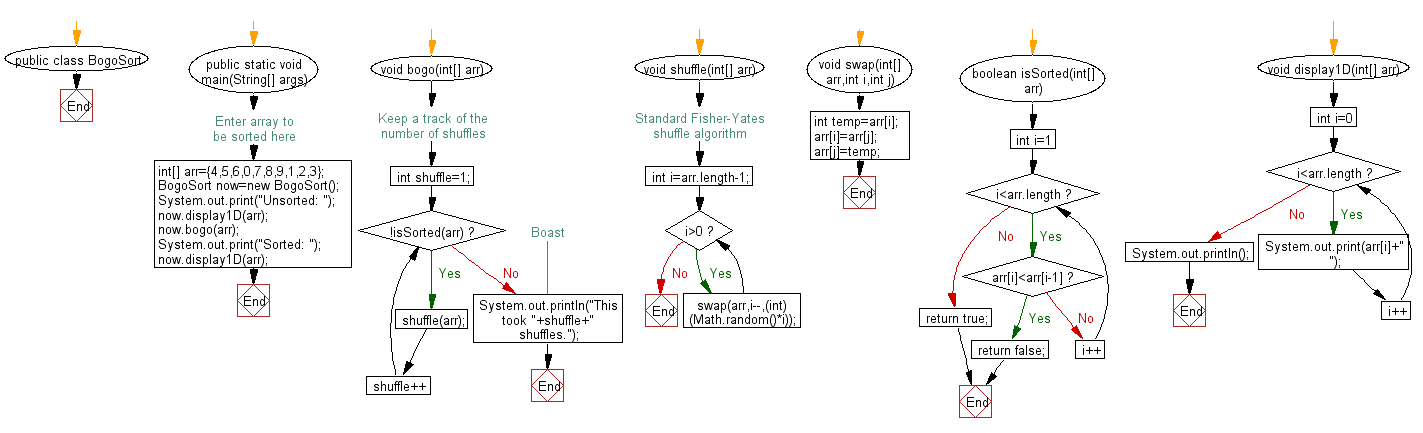﻿ Java exercises: BogoSort Sort Algorithm - w3resource# Java Exercises: BogoSort Sort Algorithm

## Java Sorting Algorithm: Exercise-9 with Solution

Write a Java program to sort an array of positive integers using the BogoSort Sort Algorithm.

In computer science, bogosort is a particularly ineffective sorting algorithm based on the generate and test paradigm. The algorithm successively generates permutations of its input until it finds one that is sorted. It is not useful for sorting but may be used for educational purposes, to contrast it with other more realistic algorithms.

Sample Solution:

Java Code:

``````public class BogoSort
{
public static void main(String[] args)
{
//Enter array to be sorted here
int[] arr={4,5,6,0,7,8,9,1,2,3};

BogoSort now=new BogoSort();
System.out.print("Unsorted: ");
now.display1D(arr);

now.bogo(arr);

System.out.print("Sorted: ");
now.display1D(arr);
}
void bogo(int[] arr)
{
//Keep a track of the number of shuffles
int shuffle=1;
for(;!isSorted(arr);shuffle++)
shuffle(arr);
//Boast
System.out.println("This took "+shuffle+" shuffles.");
}
void shuffle(int[] arr)
{
//Standard Fisher-Yates shuffle algorithm
int i=arr.length-1;
while(i>0)
swap(arr,i--,(int)(Math.random()*i));
}
void swap(int[] arr,int i,int j)
{
int temp=arr[i];
arr[i]=arr[j];
arr[j]=temp;
}
boolean isSorted(int[] arr)
{

for(int i=1;i<arr.length;i++)
if(arr[i]<arr[i-1])
return false;
return true;
}
void display1D(int[] arr)
{
for(int i=0;i<arr.length;i++)
System.out.print(arr[i]+" ");
System.out.println();
}
}

``````

Sample Output:

```Unsorted: 4 5 6 0 7 8 9 1 2 3
This took 3067022 shuffles.
Sorted: 0 1 2 3 4 5 6 7 8 9
```

Flowchart:Java Code Editor:

What is the difficulty level of this exercise?

﻿

## Java: Tips of the Day

Array vs ArrayLists:

The main difference between these two is that an Array is of fixed size so once you have created an Array you cannot change it but the ArrayList is not of fixed size. You can create instances of ArrayLists without specifying its size. So if you create such instances of an ArrayList without specifying its size Java will create an instance of an ArrayList of default size.

Once an ArrayList is full it re-sizes itself. In fact, an ArrayList is internally supported by an array. So when an ArrayList is resized it will slow down its performance a bit as the contents of the old Array must be copied to a new Array.

At the same time, it's compulsory to specify the size of an Array directly or indirectly while creating it. And also Arrays can store both primitives and objects while ArrayLists only can store objects.

Ref: https://bit.ly/3o8L2KH